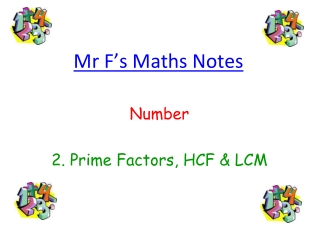DownloadDownload PresentationMr F’s Maths Notes

# Mr F’s Maths Notes

Télécharger la présentation## Mr F’s Maths Notes

- - - - - - - - - - - - - - - - - - - - - - - - - - - E N D - - - - - - - - - - - - - - - - - - - - - - - - - - -
##### Presentation Transcript

1. Mr F’s Maths Notes Number 2. Prime Factors, HCF & LCM

2. 1. Prime Factors Any positive integer can be written as a product of its prime factors. Now, that may sound complicated, but all it means is that you can break up any number into a multiplication of prime numbers, and it’s really easy to do with Factor Trees! Don’t Forget:1 is NOT a prime number, so will NEVER be in your factor tree e.g.Express 60 as a product of its prime factors 2. Prime Factors, HCF & LCM 60 60 You can break the number up however you like: 6 x 10 or 12 x 5 6 10 12 5 x x Continue breaking up each new number into a multiplication 3 2 2 5 3 4 x x x Stop when you reach a Prime Number and put a ring around it 2 x 2 3 x 2 x 2 x 5 = 60 Check your answer by multiplying all the numbers together 3 x 2 x 2 x 5 = 60

3. Look: Even though we started a different way, we still ended up with the same answer! Now, it looks good if you write your answer starting with the smallest numbers: So:60 = 2 x 2 x 3 x 5 And if you want to be really posh, you can useindices: So:60 = 22 x 3 x 5 Now we’ll do a harder one, but the technique is just the same. e.g.Express 360 as a product of its prime factors 360 You can break the number up however you like. I just went for 36 x 10 because it was easy to spot Continue breaking up each new number into a multiplication 36 10 x Stop when you reach a Prime Number and put a ring around it 6 6 2 5 x x Check your answer by multiplying all the numbers together 2 x 2 x 3 3 Write the numbers in order 3 x 2 x 3 x 2 x 2 x 5 = 360 If you can, use indices 360 = 2 x 2 x 2 x 3 x 3 x 5 360 = 23 x 32 x 5

4. 2. Highest Common Factor The Highest Common Factor (HCF) of two numbers, is the highest number that divides exactly into both 3. Lowest Common Multiple The Lowest Common Multiple (LCM) of two numbers, is the lowest number that is in the times table of both your numbers Now, you can find both of these by trial and error, but I will show you a better way! e.g.Find the LCM and HCF of 24 and 40 First, use Factor Trees to express your numbers as products of their prime factors: 24 40 12 2 4 10 x x 6 2 2 2 2 5 x x x 24 = 2 x 2 x 2 x 3 40 = 2 x 2 x 2 x 5 3 2 x

5. Now, write your answers on top of each other, like this: 24 = 2 x 2 x 2 x 3 40 = 2 x 2 x 2 x 5 Draw two inter-locking circles, and label one 24 and the other 40 24 40 Any numbers that appear in both answers go in the middle (the three 2s). The numbers left over go in the circle they belong to 2 3 5 2 2 Now, here is the clever bit: To get the Highest Common Factor you just multiply all the numbers in the middle So,HCF = 2 x 2 x 2 = 8 To get the Lowest Common Multiple you just multiply every number you can see So,LCM = 3 x 2 x 2 x 2 x 5 = 120

6. Good luck with your revision!